Function Repository Resource:

# ReverseAccumulate

Replace each element of a list with the sum of the elements in the original list that are not to the left of that element

Contributed by: Seth J. Chandler
 ResourceFunction["ReverseAccumulate"][list] computes the successive accumulated totals of list, proceding from right to left.

## Details and Options

ResourceFunction["ReverseAccumulate"] is efficiently computed by reversing the original list, running Accumulate on it and then reversing the result.
ResourceFunction["ReverseAccumulate"] is useful in computing actuarial commutation functions.

## Examples

### Basic Examples (2)

Get the sum of elements not to the left of each element in a list:

 In:=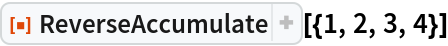Out=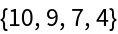Apply ReverseAccumulate to a symbolic array:

 In:=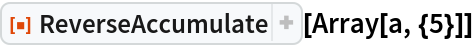Out=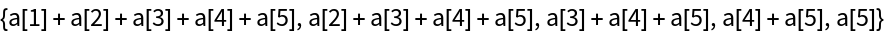### Scope (3)

Actuarial computations sometimes depend on nested ReverseAccumulate operations:

 In:=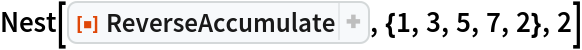Out=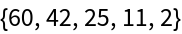The Head of an expression does not need to be List for ReverseAccumulate to work:

 In:=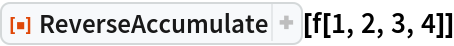Out=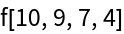Where the argument is a nested list, X, ReverseAccumulate creates a nested list Y of similar dimension in which Y[[i,j]]=ReverseAccumulate[X[[All,j]]][[i]]:

 In:=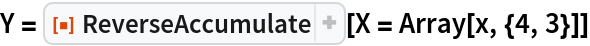Out=In:=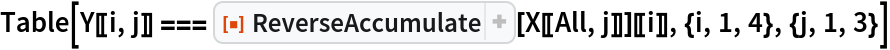Out=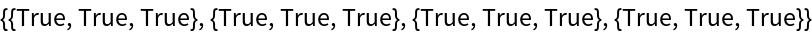### Applications (1)

Compute the values of the actuarial commutation function S for a survival list and an annual discount value of 0.8:

 In:=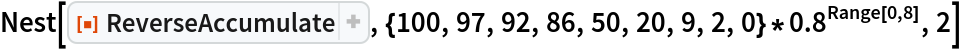Out=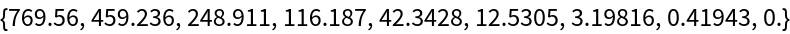### Properties and Relations (2)

The ReverseAccumulate of a list is equivalent to the Reverse of the Accumulate of the Reverse of the list:

 In:=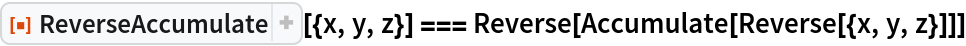Out=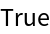One can generalize the idea of ReverseAccumulate to include operations that do not involve addition:

 In:=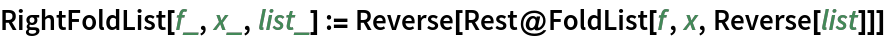In:=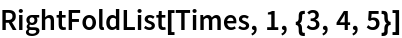Out=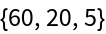Indeed, ReverseAccumulate is a special case of the following RightFoldList:

 In:=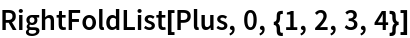Out=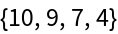Seth J. Chandler

## Version History

• 1.0.0 – 10 September 2019